Next: Universal dendrites of order Up: Dendrites Previous: Universal dendrites

## Wazewski universal dendrite

By the Wazewski universal dendrite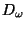we mean a dendritesuch that each ramification point ofis of order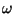and for each arc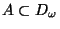the set of ramification points ofwhich belong to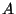is dense in. Its construction, known from [Whyburn 1942, Chapter K, p. 137] (compare also [Menger 1932, Chapter X, Section 6, p. 318]), is the following.

Let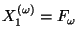. At the midpoint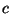of each maximal free arc contained in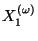(obviously the arc is a straight line segment) attach a sufficiently small copy of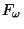so thatis the only common point ofand of the attached copy. Denote by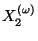the union ofand of all attached copies. Thusis a dendrite. At the midpoint of each maximal free arc contained inwe perform the same construction, i.e., we attach a sufficiently small copy ofso that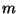is the only common point ofand of the attached copy. Denote by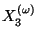the union ofand of all attached copies. Thusis a dendrite. Continuing in this way we obtain an increasing sequence of dendrites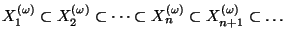. The construction can be done in the plane in such a way that the limit continuumdefined by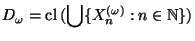is again a dendrite. See Figure A.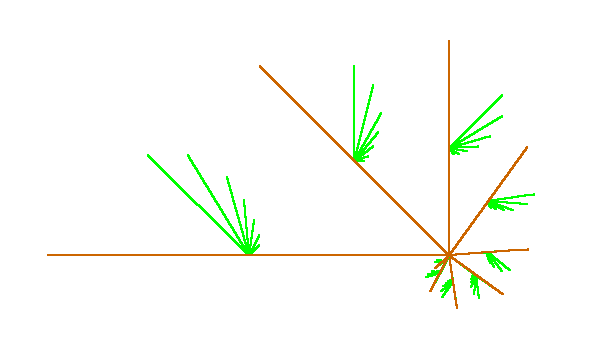For another construction of(using inverse limits) see [Nadler 1992, 10.37, p. 181-185].

The following properties ofare known.

1.is universal in the class of all dendrites (see e.g. [Nadler 1992, 10.37, p. 181-185]).
2.is embeddable in the plane (in fact, it is constructed in the plane).
3. Each open image ofis homeomorphic to(see [Chaaratonik 1980, Theorem 1, p. 490]).
4.is homogeneous with respect to monotone mappings, [Charatonik 1991, Theorem 7.1, p. 186].

For other mapping properties of, in particular ones related to the action of the group of autohomeomorphisms on, see [Charatonik 1995].

Here you can find source files of this example.

Here you can check the table of properties of individual continua.

Here you can read Notes or write to Notes ies of individual continua.Next: Universal dendrites of order Up: Dendrites Previous: Universal dendrites
Janusz J. Charatonik, Pawel Krupski and Pavel Pyrih
2001-11-30